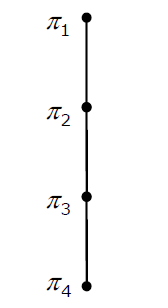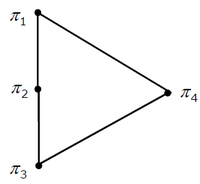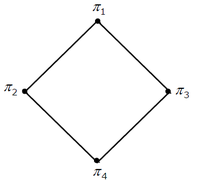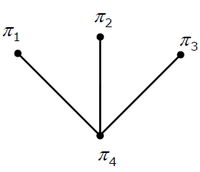# GATE | GATE-CS-2007 | Question 26

Consider the set S = (A, B, C, D}. Consider the following 4 partitions π1, π2, π3, π4 on S : π1 = {}, π2 = {}, π3 = {}, π4 = {}. Let P be the partial order on the set of partitions S’ = {π1, π2, π3, π4} defined as follows : πi P πj if and only if πi refines πj. The poset diagram for (S’, P ) is :

(A)(B)(C)(D)Explanation:

A partition is said to refine another partition if it splits the sets in the second partition to a larger number of sets.
Corresponding Hasse diagram is Option C.

Quiz of this Question
Please comment below if you find anything wrong in the above post